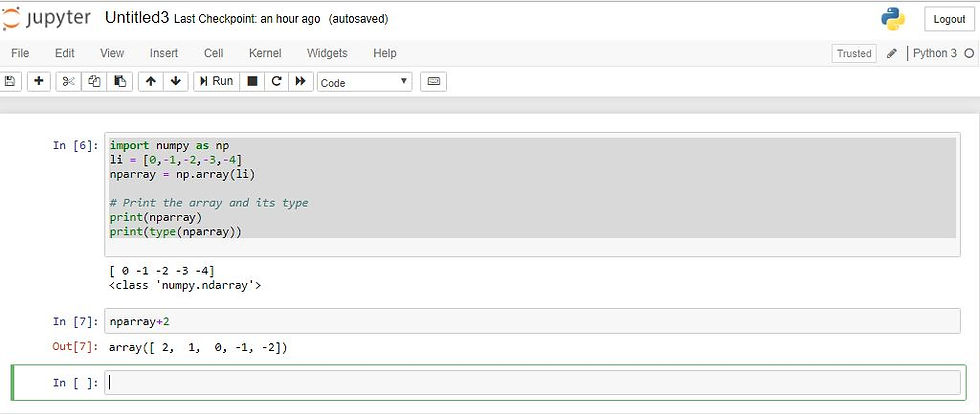top of page
Search

# Data Science Homework Help - NumPyData Science Homework Help - NumPy

In this blog, we will learn all Data Science NumPy related topics which help to become a effective data scientist. Now a day data is very useful to find important information which help to growth the any data science related industry.

Here we will include top rated example, which help to learn python.

### What is NumPy?

Numpy is the basic and a very powerful package for mathematical calculation working with data in python.

It is a Python library that provides a multidimensional array object, various derived objects (such as masked arrays and matrices).

It provides ndarray objects. By using 'ndarray' also known as array used to store multiple item of same data type.

### Characteristics of "NumPy"

Here, NumPy is has many characteristics which is given below -

• NumPy arrays have a fixed size at creation

• The elements in a NumPy array are all required to be of the same data type, and thus will be the same size in memory

• NumPy arrays facilitate advanced mathematical and other types of operations on large numbers of data.

### How to import NumPy

import numpy as np

### Creating a "NumPy" array

There are multiple ways to create NumPy but here we will learn one simple and currently useful way to create NumPy array.

Example:

### "Jupyter notebook" output### Difference between "list" and "ndarray"

There are some minor differences between list and ndarray is that

1- ndarray perform vector operations but list does not perform it, means you can performed function at every item of array but not in list.

for example:

if you want to add something to the list-

>>> li+2 # show error

then it show error but in ndarray you can add 2 at every item of array.

>>> nparray+2 # where nparray take from example

Example:2- size of ndarray is fixed and can not be change after it is created to do this another array is created but in list size is extended.

• float

• int

• bool

• str

• object

### Control the memory allocations

To control memory allocations use of one which is given below -

float32, float64, int8, ‘int16, int32

Example: first creating create 2D array using list of list

Output by jupyter notebook:Output with float and int: Convert to "int" and "float" data type3- In numpy all item is same data type unlike list.

Example:

Output by jupyter notebook:### Array convert back to list

We can create array back to list - use tolist() to convert back to array

Example:

Output:### Action performed with array

In this we will performed some some important operation with array as -

Extracting row and column -

It start from index 0

Example:But using list it show error -### numpy arrays support boolean indexing

Give boolean output when condition check -

For Example:

np = nparray > 4

Output using Jupyter notebook:### Reverse the rows and the whole array

In numpy we can reverse the rows of whole array -

nparray[::-1, ] #reverse only the row position

nparray[::-1, ::-1] #reverse both row and column position

### Compute mean, min, max

Use these functions to find mean, min and max

• nparray.mean()

• nparray.max()

• nparray.min()

Find min and max values row wise or column wise

• np.amin(nparray, axis=0) # column wise minimum

• np.amin(nparray, axis=1) # row wise minimum

### Create a new array from an existing

You can also create array from an existing array in python numpy

### Reshaping an array m×n to n×m shape

Array can be reshape in python numpy easily

Use reshape(n,m) functions

Example:

nparray.reshape(n,m)

### Creating sequences using python numpy

To create sequence using python numpy.

We use arange(5) function to generate sequence.

Example:

nparray.arange(5)

Output look like that

[0, 1, 2, 3, 4, 5]

With step(gap):

nparray.arange(0, 9) # from 0 to 9

nparray.arange(0, 9, 2) # from 0 to 9 with gap 2

nparray.arange(0, 9, -1) # Decresing order

On working.....it is on working

Other Data Science related blogs:

If you like Codersarts blog and looking for Programming Assignment Help Service,Database Development Service,Web development service,Mobile App Development, Project help, Hire Software Developer,Programming tutors help and suggestion  you can send mail at contact@codersarts.com.

Please write your suggestion in comment section below if you find anything incorrect in this blog post

bottom of page